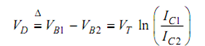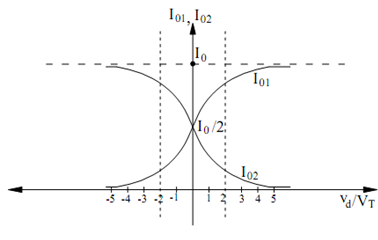## Linear amplifier Assignment Help

Assignment Help: >> Differential Amplifier - Linear amplifier

Linear amplifier:

In the following, we carry out a DC analysis of the circuit, to ascertain the range of operation over which it acts as a linear amplifier.

Supposing Q1 ≅ Q2 and β1,2 >> 1 so that we can assume IE1, 2 ≅ IC 1, 2, the application of KVL to the base loop gives

VB1 = VBE1 - VBE 2  + VB 2

Under normal operating condition, according to the Ebers-Moll equations, we have

Ic  ≅ I s  e (VBE /VT)

where Is stands for the reverse saturation current and VT = kT/q. Therefore,

V BE      = V T ln (   IC  /IS)

From Eqs. (34) and (36), we have

VB1 - VB2 = VT ln [  ( IC1  /IS2)  /  ( IC1  /IS1)

For matched transistors IS1 = IS2 so that, we geton the other hand, one can write

IC1 / IC 2= e (VD /VT)

Since,

IC1 + IC 2  ≅ I E1 + I E 2  = I EE  = I0

Solving Eqs. (39) and (40) for IC1 and IC2 we obtain

I01 = IC1  = I0 /(1+  e (- VD  / VT )  )

and

I02 = IC 2 =  I0 /1 + e+ (VD/ VT  )

Figure illustrates a plot of these two equations. From the plot we can note that when VD

= 0, i.e. VB1 = VB2, IC1 = IC2 = IEE /2 which is an expected result due to the supposition of matched devices. Also, for VD /VT > 4, IC1 → IEE and IC2 → 0; likewise, for VD /VT → 4, IC1 → 0 and IC2 → IEE. Under either of these two conditions, one of the transistors is saturated and the other is cutoff.Figure#### Assured A++ Grade

Get guaranteed satisfaction & time on delivery in every assignment order you paid with us! We ensure premium quality solution document along with free turntin report!

All rights reserved! Copyrights ©2019-2020 ExpertsMind IT Educational Pvt Ltd